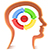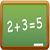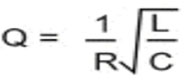PART66 Licensing Info
Licence Categories
Modularization
Number of questions
Experience requirements
Licence extension

Remember me

Registration
Module:     Show:Module 9. Human factors
An engineer is working on a wing and kicks a spanner off into an engine cowl and breaks a sensor. This is
•  knowledge based error.
•  skill based error.
•  rule based error.Module 1. Mathematics
Find L in the following expression:•  L = Q^2 * C^2 / R
•  L = Q^2 * C / R^2
•  L = Q^2 * R^2 * CModule 1. Mathematics
Make m the subject of the formula in y=mx+c.
•  m=(y-c)/x
•  m=y-x/c
•  m=y+c/xModule 1. Mathematics
Solve the simultaneous equations y = x + 2 and 3y + 2x = 6.
•  x = 0, y = 0
•  x = 0, y = 2
•  x = 2, y = 0Module 15. Gas turbine engine
If a burner was down, in a multi-can system, the engine would tend to
•  hang up.
•  run up.
•  surge.